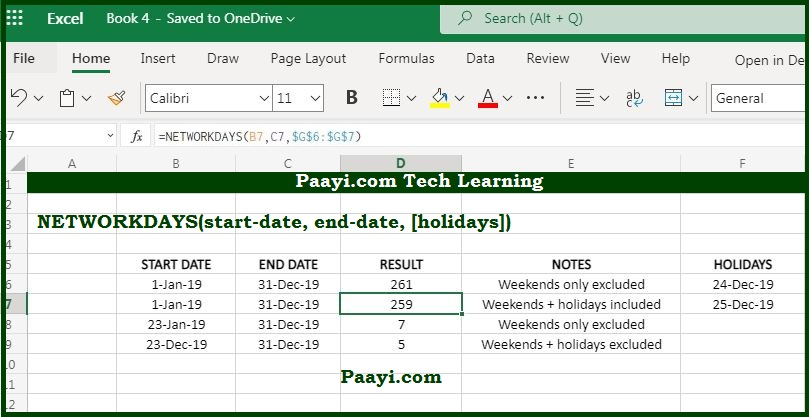# Learn How to Use Microsoft Excel NETWORKDAYS Function

Written by | 0 Comments | 727 Views

In this article, you will learn how to use the Microsoft Excel NETWORKDAYS function and its prime function in Microsoft Excel. You will also get to know the Microsoft Excel NETWORKDAYS function return value and syntax with the help of some examples.

## Microsoft Excel NETWORKDAYS Function

The Microsoft Excel NETWORKDAYS function gets the number of working days between the two specified dates. That means the NETWORKDAYS function is used to calculate the number of working days between the two dates. NETWORKDAYS function automatically excludes Saturdays and Sundays, and you also have an option to exclude the list of holidays that are provided as dates.

NETWORKDAYS function includes also includes the start date and end date, which are specified while calculating the working days. As an option, the NETWORKDAYS function can also take care of supplied holidays; for these holidays, you need to provide dates containing the holidays and treat them as non-working days.

### NETWORKDAYS Function Return Value

The number representing working days.

### NETWORKDAYS Syntax

= NETWORKDAYS(start-date, end-date, [holidays])

Where:

• start-date: The start date
• end-date: The end date
• holidays: A list of working days supplied as holidays (optional).

## How to Use Microsoft Excel NETWORKDAYS Function?As we know, the NETWORKDAYS function is used to calculate the working days between the two specified dates and also exclude the list of supplied holidays.

Example 1: Let's say, for example, the start date in A1 and end date is in B1; you can use the NETWORKDAYS function as given below:

• = NETWORKDAYS(A1, B1) - Will return the total number of working days between the two specified dates.

Example 2: You can also exclude the holidays, which you can supply in the formula as a list of dates in C1:C5:

• = NETWORKDAYS(A1, B1, C1:C5) - This will return the total number of working days, also excluding the supplied list of the holidays.